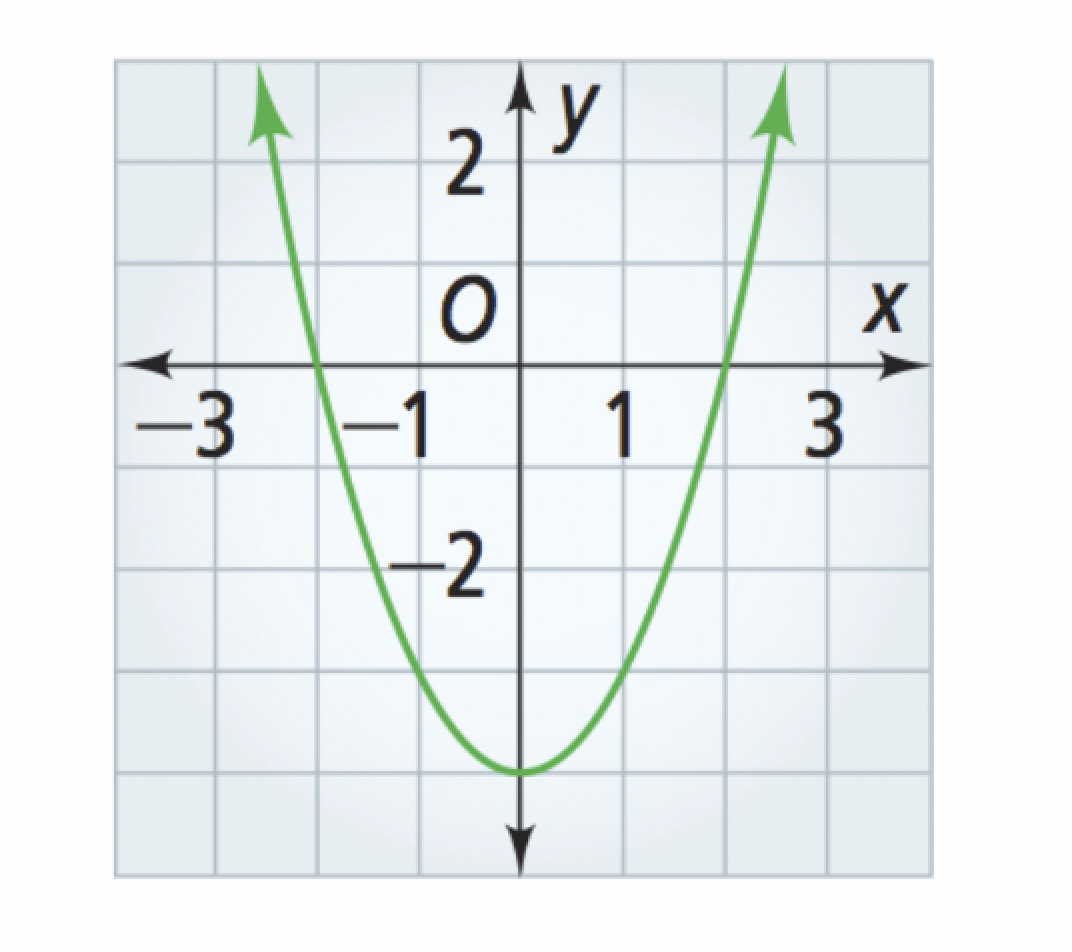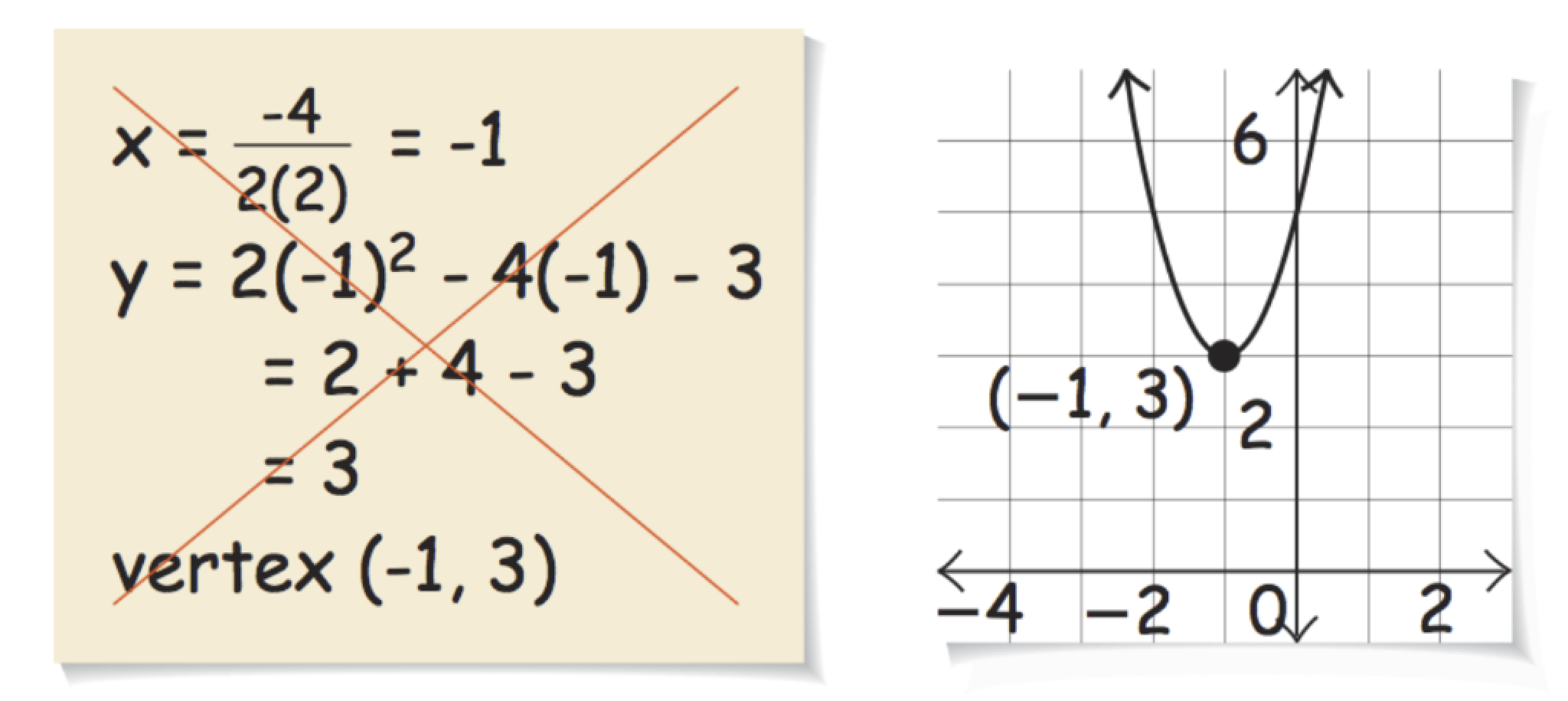Algebra 2 4-2 Independent Practice: Standard Form of a Quadratic Function
starstarstarstarstarstarstarstarstarstar
by Matthew Richardson
| 9 Questions1
1
10
Vocabulary: Identify the vertex of the parabola.
(-2, 0)
y = 3x - 2
y = 0
(0, -4)
x = 0
(2, 0)2
2
10
Vocabulary: Identify the axis of symetry of the parabola.
(-2, 0)
y = 3x - 2
y = 0
(0, -4)
x = 0
(2, 0)3
3
10
Vocabulary: Identify the minimum of the parabola.
(-2, 0)
y = 3x - 2
y = 0
(0, -4)
x = 0
(2, 0)4
4
5
Vocabulary: Does the parbola have a maximum?
Yes
No
5
20
Graphing: Graph the function. Sketch the function yourself before using a graphing utility, like the embedded Desmos graphing calculator, to check your work. Be sure to include relevant graph detail: label axes, indicate units and scale on both axes, and use arrows to represent end behavior, as appropriate.

6
20
Graphing: Graph the function. Sketch the function yourself before using a graphing utility, like the embedded Desmos graphing calculator, to check your work. Be sure to include relevant graph detail: label axes, indicate units and scale on both axes, and use arrows to represent end behavior, as appropriate.7
7
10
Error Analysis: A student graphed the function below as shown in the image.
Find and correct the error.
9
10
Reflection: Math Success